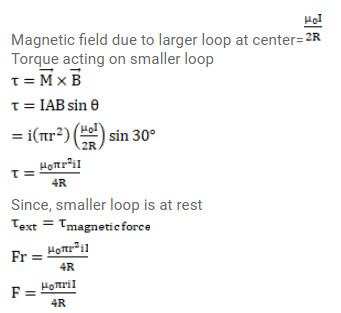# A circular loop of radius r carrying a current i is held

Question:

A circular loop of radius $r$ carrying a current $i$ is held at the center of another circular loop of radius $R$ ( $>r$ ) carrying a current I. The plane of the smaller loop makes an angle of $30^{\circ}$ with that of the larger loop. If the smaller loop is held fixed in this position by applying a single force at a point on its periphery, what would be the minimum magnitude of this force?

Solution: